Next: 3-Dimensional Quantum Gravity Up: Planck Previous: The Planck Length

# 3. Topological Quantum Field Theory

Besides general relativity and quantum field theory as usually practiced, a third sort of idealization of the physical world has attracted a great deal of attention in the last decade. These are called topological quantum field theories, or TQFTs'. In the terminology of the previous section, a TQFT is a background-free quantum theory with no local degrees of freedom1.

A good example is quantum gravity in 3-dimensional spacetime. First let us recall some features of classical gravity in 3-dimensional spacetime. Classically, Einstein's equations predict qualitatively very different phenomena depending on the dimension of spacetime. If spacetime has 4 or more dimensions, Einstein's equations imply that the metric has local degrees of freedom. In other words, the curvature of spacetime at a given point is not completely determined by the flow of energy and momentum through that point: it is an independent variable in its own right. For example, even in the vacuum, where the energy-momentum tensor vanishes, localized ripples of curvature can propagate in the form of gravitational radiation. In 3-dimensional spacetime, however, Einstein's equations suffice to completely determine the curvature at a given point of spacetime in terms of the flow of energy and momentum through that point. We thus say that the metric has no local degrees of freedom. In particular, in the vacuum the metric is flat, so every small patch of empty spacetime looks exactly like every other.

The absence of local degrees of freedom makes general relativity far simpler in 3-dimensional spacetime than in higher dimensions. Perhaps surprisingly, it is still somewhat interesting. The reason is the presence of global' degrees of freedom. For example, if we chop a cube out of flat 3-dimensional Minkowski space and form a 3-dimensional torus by identifying the opposite faces of this cube, we get a spacetime with a flat metric on it, and thus a solution of the vacuum Einstein equations. If we do the same starting with a larger cube, or a parallelipiped, we get a different spacetime that also satisfies the vacuum Einstein equations. The two spacetimes are locally indistinguishable, since locally both look just like flat Minkowski spacetime. However, they can be distinguished globally -- for example, by measuring the volume of the whole spacetime, or studying the behavior of geodesics that wrap all the way around the torus.

Since the metric has no local degrees of freedom in 3-dimensional general relativity, this theory is much easier to quantize than the physically relevant 4-dimensional case. In the simplest situation, where we consider pure' gravity without matter, we obtain a background-free quantum field theory with no local degrees of freedom whatsoever: a TQFT.

I shall say more about 3-dimensional quantum gravity in Section 4. To set the stage, let me sketch the axiomatic approach to topological quantum field theory proposed by Atiyah . My earlier definition of a TQFT as a background-free quantum field theory with no local degrees of freedom' corresponds fairly well to how physicists think about TQFTs. But mathematicians who wish to prove theorems about TQFTs need to start with something more precise, so they often use Atiyah's axioms.

An important feature of TQFTs is that they do not presume a fixed topology for space or spacetime. In other words, when dealing with an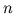-dimensional TQFT, we are free to choose any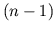-dimensional manifold to represent space at a given time2. Moreover given two such manifolds, say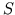and, we are free to choose any-dimensional manifold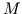to represent the portion of spacetime betweenand. Mathematicians calla cobordism' fromto. We write, because we may think ofas the process of time passing from the momentto the moment.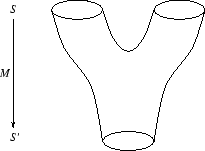For example, in Figure 1 we depict a 2-dimensional manifoldgoing from a 1-dimensional manifold(a pair of circles) to a 1-dimensional manifold(a single circle). Crudely speaking,represents a process in which two separate spaces collide to form a single one! This may seem outré, but these days physicists are quite willing to speculate about processes in which the topology of space changes with the passage of time. Other forms of topology change include the the formation of a wormhole, the appearance of the universe in a big bang', or its disappearance in a big crunch'.

There are various important operations one can perform on cobordisms, but I will only describe two. First, we may compose' two cobordismsand, obtaining a cobordism, as illustrated in Figure 2. The idea here is that the passage of time corresponding tofollowed by the passage of time corresponding to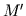equals the passage of time corresponding to. This is analogous to the familiar idea that waiting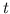seconds followed by waitingseconds is the same as waiting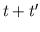seconds. The big difference is that in topological quantum field theory we cannot measure time in seconds, because there is no background metric available to let us count the passage of time! We can only keep track of topology change. Just as ordinary addition is associative, composition of cobordisms satisfies the associative law: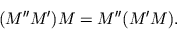However, composition of cobordisms is not commutative. As we shall see, this is related to the famous noncommutativity of observables in quantum theory.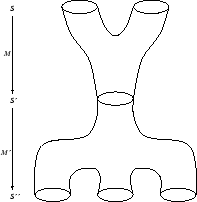Second, for any-dimensional manifoldrepresenting space, there is a cobordismcalled the identity' cobordism, which represents a passage of time without any topology change. For example, whenis a circle, the identity cobordism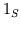is a cylinder, as shown in Figure 3. In general, the identity cobordismhas the property that for any cobordismwe have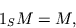while for any cobordismwe haveThese properties say that an identity cobordism is analogous to waiting 0 seconds: if you wait 0 seconds and then waitmore seconds, or waitseconds and then wait 0 more seconds, this is the same as waitingseconds.These operations just formalize of the notion of the passage of time' in a context where the topology of spacetime is arbitrary and there is no background metric. Atiyah's axioms relate this notion to quantum theory as follows. First, a TQFT must assign a Hilbert space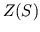to each-dimensional manifold. Vectors in this Hilbert space represent possible states of the universe given that space is the manifold. Second, the TQFT must assign a linear operator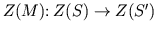to each-dimensional cobordism. This operator describes how states change given that the portion of spacetime betweenandis the manifold. In other words, if space is initially the manifoldand the state of the universe is, after the passage of time corresponding tothe state of the universe will be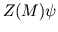.

In addition, the TQFT must satisfy a list of properties. Let me just mention two. First, the TQFT must preserve composition. That is, given cobordismsand, we must havewhere the right-hand side denotes the composite of the operatorsand. Second, it must preserve identities. That is, given any manifoldrepresenting space, we must have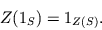where the right-hand side denotes the identity operator on the Hilbert space.

Both these axioms are eminently reasonable if one ponders them a bit. The first says that the passage of time corresponding to the cobordismfollowed by the passage of time corresponding tohas the same effect on a state as the combined passage of time corresponding to. The second says that a passage of time in which no topology change occurs has no effect at all on the state of the universe. This seems paradoxical at first, since it seems we regularly observe things happening even in the absence of topology change. However, this paradox is easily resolved: a TQFT describes a world quite unlike ours, one without local degrees of freedom. In such a world, nothing local happens, so the state of the universe can only change when the topology of space itself changes3.

The most interesting thing about the TQFT axioms is their common formal character. Loosely speaking, they all say that a TQFT maps structures in differential topology -- by which I mean the study of manifolds -- to corresponding structures in quantum theory. In coming up with these axioms, Atiyah took advantage of a powerful analogy between differential topology and quantum theory, summarized in Table 1.

 DIFFERENTIAL TOPOLOGY QUANTUM THEORY-dimensional manifold (space) Hilbert space (states) cobordism between-dimensional manifolds (spacetime) operator (process) composition of cobordisms composition of operators identity cobordism identity operator

Table 1: Analogy between differential topology and quantum theory

I shall explain this analogy between differential topology and quantum theory further in Section 5. For now, let me just emphasize that this analogy is exactly the sort of clue we should pursue for a deeper understanding of quantum gravity. At first glance, general relativity and quantum theory look very different mathematically: one deals with space and spacetime, the other with Hilbert spaces and operators. Combining them has always seemed a bit like mixing oil and water. But topological quantum field theory suggests that perhaps they are not so different after all! Even better, it suggests a concrete program of synthesizing the two, which many mathematical physicists are currently pursuing. Sometimes this goes by the name of quantum topology' [3,30].

Quantum topology is very technical, as anything involving mathematical physicists inevitably becomes. But if we stand back a moment, it should be perfectly obvious that differential topology and quantum theory must merge if we are to understand background-free quantum field theories. In physics that ignores general relativity, we treat space as a background on which states of the world are displayed. Similarly, we treat spacetime as a background on which the process of change occurs. But these are idealizations which we must overcome in a background-free theory. In fact, the concepts of space' and state' are two aspects of a unified whole, and likewise for the concepts of spacetime' and `process'. It is a challenge, not just for mathematical physicists, but also for philosophers, to understand this more deeply.

Next: 3-Dimensional Quantum Gravity Up: Planck Previous: The Planck Length